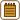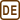## Heat Conduction

### Task number: 2177

Space between two parallel plates is filled with air under normal conditions. Area of each plate is 100 cm2 and distance between the plates is 1 mm. Temperature difference between the plates is maintained constant and equal to 1 K. Determine the amount of heat transferred from one of the plates to the other one in 10 minutes due to the thermal conductivity of air.

Assume that the diameter of an air molecule is approximately 0.3 nm and specific heat capacity of air is as follows

$c_{V}\,=\,\frac{5R}{2M_m},$

where Mm stands for the molar mass of air. Density of air is 1.293 kg m−3.

• #### Hint 1

It is said in the task assignment that the described process takes place under normal conditions. What does it mean?

• #### Hint 2: Calculating the Heat

To calculate the heat Q transferred between two plates in time τ, use the so-called Fourier's law

$Q=\frac{\lambda A \mathrm{\Delta}T\tau}{l},$

where l is the distance between the plates, A area of the plates, ΔT temperature difference between the plates (the relation above holds true only if the temperature difference is constant throughout the process) and λ is the thermal conductivity coefficient.

We know the values of all quantities used in the formula except the thermal conductivity coefficient. Think (look up) how it can be determined.

• #### Notation

 A = 100 cm2 = 0.01 m2 area of each plate l = 1 mm = 0.001 m distance between the plates ΔT = 1 K temperature difference between the plates τ = 10 min = 600 s duration of heat transfer d = 0.3 nm = 3·10−10 m diameter of an air “molecule” ρ = 1.293 kg m−3 density of air Q = ? transferred heat

From the Tables:

 Mm = 0.029 kg mol−1 molar mass of air T = 273.15 K temperature under normal conditions p = 101 325 Pa pressure under normal conditions R = 8.31 JK−1mol−1 molar gas constant k = 1.38·10−23 JK−1 Boltzmann constant
• #### Analysis

To calculate the conducted heat, we use Fourier's law of thermal conduction. According to this law, transferred heat is directly proportional to the coefficient of thermal conductivity, area of the plates, temperature difference between the plates and duration of the process, and inversely proportional to the distance between the plates.

Coefficient of thermal conductivity is determined by the mean molecular speed and mean free path.

• #### Solution

Heat Q transferred between two plates during time τ can be determined from Fourier's law of heat conduction as follows

$Q=\frac{\lambda A \mathrm{\Delta}T\tau}{l},$

where l is the distance between the plates, A area of the plates, ΔT temperature difference between the plates (the relation above holds true only if the temperature difference is constant throughout the process) and λ is the thermal conductivity coefficient.

Coefficient of thermal conductivity is the only quantity, whose value is not given in the task assignment. However, we can calculate this coefficient using the kinetic theory of gases, which provides us with the following relation between the thermal conductivity λ, mean molecular speed $$\bar{v}$$, mean free path $$\bar{\lambda}$$, density of the gas ρ and specific heat capacity of the gas cv:

$\lambda=\frac{1}{2}\bar{v}\bar{\lambda}\rho c_{v}.$

Mean molecular speed $$\bar{v}$$ can be determined from the following relation

$\bar{v}=\sqrt{\frac{8RT}{\pi M_{m}}},$

where R is the gas constant, T temperature under normal conditions and Mm molar mass of air.

Mean free path $$\bar{\lambda}$$ can be calculated from the formula

$\bar{\lambda}=\frac{kT}{p d^{2}\pi \sqrt{2}},$

where k is Boltzmann constant, p pressure under normal conditions and d diameter of the air “molecule”.

Using these two formulae above and the relation for the specific heat capacity of air, we obtain the formula for the coefficient of thermal conductivity λ

$\lambda=\frac{1}{2}\,\bar{v}\bar{\lambda}\rho c_{v}=\frac{1}{2}\sqrt{\frac{8RT}{\pi M_{m}}}\,\frac{kT}{p d^{2}\pi \sqrt{2}}\,\rho\,\frac{5R}{2M_{m}}.$

Adjusting the formula, we get

$\lambda=\frac{5}{2}\,\left(\frac{RT}{\pi M_{m}}\right)^{\frac{3}{2}}\,\frac{k\rho}{pd^{2}}.$

By substituting the coefficient of thermal conductivity in the relation for the transferred heat Q, we obtain the final formula

$Q=\frac{\lambda A \mathrm{\Delta}T\tau}{l}\,=\,\frac{5}{2}\,\left(\frac{RT}{\pi M_{m}}\right)^{\frac{3}{2}}\,\frac{k\rho}{pd^{2}}\,\frac{A \mathrm{\Delta}T\tau}{l}.$
• #### Numerical Substitution

$Q=\frac{5}{2}\,\left(\frac{RT}{\pi M_{m}}\right)^{\frac{3}{2}}\,\frac{k\rho}{pd^{2}}\,\frac{A \mathrm{\Delta}T\tau}{l}$ $Q=\frac{5}{2}\,\left(\frac{8.31\cdot{273.15}}{\pi\cdot{ 0.029}}\right)^{\frac{3}{2}}\cdot\frac{1.38\cdot{10^{-23}}\cdot1.293}{101\,325\cdot{3^{2}}\cdot{10^{-20}}}\cdot\frac{0.01\cdot{ 1}\cdot{600}}{0.001}\,\mathrm{J}$ $Q\dot{=}120\,\mathrm{J}$
• #### Answer

Amount of heat transferred between the plates in 10 minutes is equal to 120 J.

• #### Comment

Thermal conduction in gases is not the dominant type of heat transfer. In gases, heat is transferred mainly by convection.

• #### Accuracy of the Solution

The constant in the formula for the coefficient of thermal conductivity is not exactly equal to 1/2 as we have stated in the task. Such number is based on an approximate theoretical calculation, whereas more detailed analysis provides a slightly different value. Therefore, it is possible that the obtained result is not identical with the actual value.